# Mathematical induction facts for kids

Kids Encyclopedia FactsMathematical induction can be informally illustrated by reference to the sequential effect of falling dominoes.

Mathematical induction is a special way of proving a mathematical truth. It can be used to prove that something is true for all the natural numbers (all the positive whole numbers). The idea is that

• Something is true for the first case
• That same thing is always true for the next case

then

• That same thing is true for every case

In the careful language of mathematics:

• State that the proof will be by induction over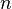$n$. ($n$ is the induction variable.)
• Show that the statement is true when$n$ is 1.
• Assume that the statement is true for any natural number$n$. (This is called the induction step.)
• Show then that the statement is true for the next number,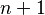$n+1$.

Because it's true for 1, then it is true for 1+1 (=2, by the induction step), then it is true for 2+1 (=3), then it is true for 3+1 (=4), and so on.

An example of proof by induction:

Prove that for all natural numbers n: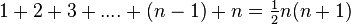$1+2+3+....+(n-1)+n=\tfrac12 n(n+1)$

Proof:

First, the statement can be written: for all natural numbers n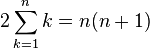$2\sum_{k=1}^n k=n(n+1)$

By induction on n,

First, for n=1: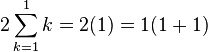$2\sum_{k=1}^1 k=2(1)=1(1+1)$,

so this is true.

Next, assume that for some n=n0 the statement is true. That is,: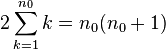$2\sum_{k=1}^{n_0} k = n_0(n_0+1)$

Then for n=n0+1: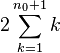$2\sum_{k=1}^{{n_0}+1} k$

can be rewritten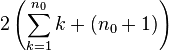$2\left( \sum_{k=1}^{n_0} k+(n_0+1) \right)$

Since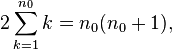$2\sum_{k=1}^{n_0} k = n_0(n_0+1),$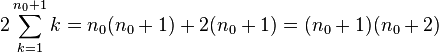$2\sum_{k=1}^{n_0+1} k = n_0(n_0+1)+2(n_0+1) =(n_0+1)(n_0+2)$

Hence the proof is correct.

## Similar proofs

Mathematical induction is often stated with the starting value 0 (rather than 1). In fact, it will work just as well with a variety of starting values. Here is an example when the starting value is 3. The sum of the interior angles of a$n$-sided polygon is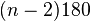$(n-2)180$degrees.

The initial starting value is 3, and the interior angles of a triangle is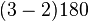$(3-2)180$degrees. Assume that the interior angles of a$n$-sided polygon is$(n-2)180$degrees. Add on a triangle which makes the figure a$n+1$-sided polygon, and that increases the count of the angles by 180 degrees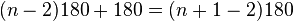$(n-2)180+180=(n+1-2)180$degrees. Proved.

There are a great many mathematical objects for which proofs by mathematical induction works. The technical term is a well-ordered set.

## Inductive definition

The same idea can work to define, as well as prove.

Define$n$th degree cousin:

• A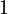$1$st degree cousin is the child of a parent's sibling
• A$n+1$st degree cousin is the child of a parent's$n$th degree cousin.

There is a set of axioms for the arithmetic of the natural numbers which is based on mathematical induction. This is called "Peano's Axioms". The undefined symbols are | and =. The axioms are

• | is a natural number
• If$n$ is a natural number, then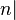$n|$ is a natural number
• If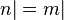$n| = m|$ then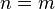$n = m$

One can then define the operations of addition and multiplication and so on by mathematical induction. For example:

•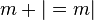$m + | = m|$
•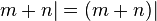$m + n| = (m + n)|$Mathematical induction Facts for Kids. Kiddle Encyclopedia.